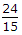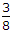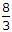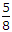# Civil Engineering - Strength of Materials

36.
The ratio of the maximum deflection of a cantilever beam with an isolated load at its free end and with a uniformly distributed load over its entire length, is
1Explanation:
No answer description is available. Let's discuss.

37.
For a beam of uniform strength keeping its depth constant, the width will vary in proportion to
bending moment (M)
M
M2
none of these.
Explanation:
No answer description is available. Let's discuss.

38.
A shaft 9 m long is subjected to a torque 30 t-m at a point 3 m distant from either end. The reactive torque at the nearer end will be
5 tonnes metre
10 tonnes metre
15 tonnes metre
20 tonnes metre
none of these.
Explanation:
No answer description is available. Let's discuss.

39.
The width b and depth d of a beam cut from a wooden cylindrical log of 100 cm diameter for maximum strength are :
b = 57.73 cm d = 81.65 cm
b = 81.65 cm d = 57.73 cm
b = 50.00 cm d = 50.00 cm
b = 40.00 cm d = 80.00 cm
b = 30.00 cm d = 60.00 cm.